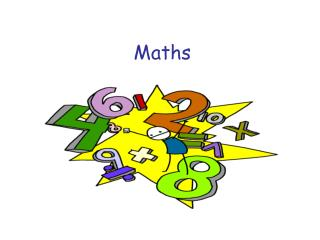DownloadDownload PresentationMaths

# Maths

Télécharger la présentation## Maths

- - - - - - - - - - - - - - - - - - - - - - - - - - - E N D - - - - - - - - - - - - - - - - - - - - - - - - - - -
##### Presentation Transcript

1. Maths

2. Questionnaire A list of questions, usually printed, submitted for replies that can be analysed for usable information; Technique used to gather information in the data handling cycle.

3. Survey To gather information for presenting data Technique used to gather information in the data handling cycle.

4. Conversion • Change in the units or form of a number or expression; The conversion of miles to kilometres involves multiplying by 1.6

5. Estimate - to form an approximate idea of (distance, size, cost, etc); calculate roughly; • Estimating length/weight of different objects.

6. Denominator - the term of a fraction usually written under the line, that indicates the number of equal parts into whichthe unit is divided; • Solving worded questions involving fractions.

7. Factor – a number that divides exactly into another number with no remainder. In the calculation of Highest Common Factor

8. Perpendicular – at right angles to; meeting a given line or surface at right angles. Properties of quadrilaterals

9. Deposit – to give (money) in part payment or as security; I paid for the car with a deposit then 24 monthly payments.

10. Quadratic – Algebra involving the square and no higher power of the unknown quantity; of the second degree. • To find two solutions to an equation = 0

11. Congruent– having identical shapes so that all parts correspond. Properties of triangles.

12. Asymptote– straight line continually approaching but never meeting a curve. Drawing of reciprocal graphs.

13. Equilibrium – a state of rest or balance due to the equal action of opposing forces. Solving real life problems to find frictional forces or co-efficient of friction.